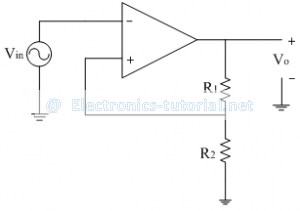Home > analog integrated circuits > schmitt trigger > inverting schmitt trigger

# Inverting Schmitt Trigger

A part of output is fed back to the non-inverting (positive) input of the op-amp, hence called as positive feedback comparator. The inverting Schmitt trigger is shown below,The triggering point VT is calculated as
VT=R2/(R1+R2 ) Vout

If Vout=+Vsat , VT=+ve
If Vout=-Vsat , VT= -ve
Thus when output is +Vsat, the upper threshold point is given as
VUT=R2/(R1+R2 ) [+Vsat ]
And when output is -Vsat, the lower threshold point is given as

VLT=R2/(R1+R2 ) [-V_sat ]

The operation of the above circuit can be explained with the two conditions
When Vin>VT ∴Vo=-Vsat
When Vin<VT ∴Vo=+Vsat
When input voltage Vin is less than upper threshold VUT, the output is in positive saturation +Vsat. When input crosses the upper threshold VUT, output is changed to negative saturation –Vsat. This output state is maintained till the next threshold level i.e. VLT. When input signal crosses the lower threshold VLT, output is changed to positive saturation.
Thus output state is changed only when the two thresholds are crossed. This is shown in the transfer characteristics. Between the VLT and VUT, output (±Vsat) remains constant i.e output is not responding to any changes in the input signal. Thus output is dead between VLT and VUT and called as dead band. It is also referred as hysteresis width, denoted by 'H'.

The input and output waveforms are shown below.Transfer characteristics:
Thus in transfer characteristics we get a rectangle. This is called as hysteresis loop. The graph indicates that the output remains in the state indefinitely until input voltage crosses the any of the threshold levels. The transfer characteristics are shown below.This hysteresis loop is also called as a dead band or dead zone because output is not changing (i.e. not responding to input signal)

The Width of Hysteresis Loop is calculated as
H=VUT – VLT
∴H= R2/(R1+R2 ) [+Vsat ]-R2/(R1+R2 ) [-Vsat ]
∴H= (2R2)/(R1+R2 ) [Vsat ]
H=2VT